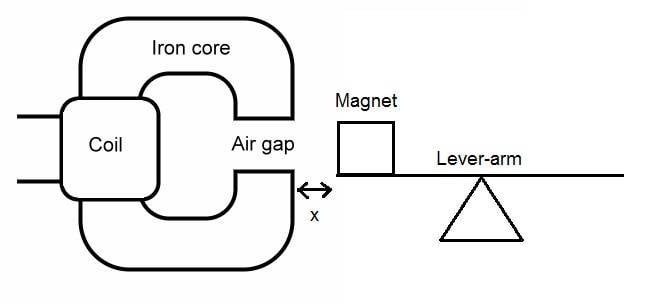# Distance variation dependency between coil and magnet

pasall
Hello!

I'm wondering if there's a general dependency/connection/correlation/function for changes in distance between an electromagnet (coil with iron core) and a magnet (Samarium Cobalt). Here's a picture to explain what I mean:If I move the coil closer to the magnet (smaller x) the force on the magnet increases and vice versa. I've discovered that it's not a linear connection. I've tried searching and the closest answer I've found is a function like f(x)=1/x^3 or f(x)=1/x^2 which I belive is called the inverese square law. However, in this case there are two magnetic fields involved so maybe it's not possible to determine such function for the above case? Any ideas?

## Answers and Replies

Gold Member
However, in this case there are two magnetic fields involved so maybe it's not possible to determine such function for the above case?
Can you determine the force if the magnet is substituted by un-magnetized iron?

When you close up the magnet/iron, the magnetic field in the air gap will be distorted, and the location at some unlinear magnetizing characteristic will change.

It will not be a 1/x2 - function, nor a 1/x3.

A computer can calculate it, using numerical calculations.

pasall
Can you determine the force if the magnet is substituted by un-magnetized iron?

Not really. I don't have the necessary equipment available and it's not that important either. Basically I wanted to know if there was a simple function/connection or if it was more complicated. I think I've got the answer (that it's very complex) and that will do just fine! Thank you for your answer!